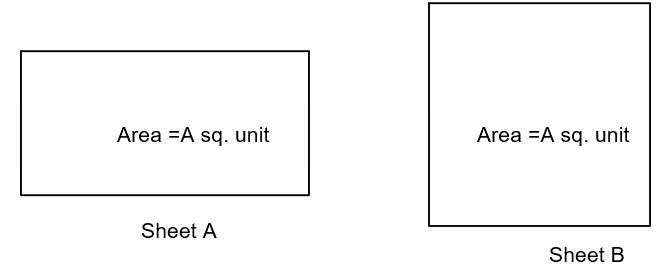Courses
Courses for Kids
Free study material
Offline Centres
MoreLast updated date: 28th Nov 2023
Total views: 280.2k
Views today: 5.80k

# Diya uses two types of sheets for painting, sheet $A$ and sheet $B$, where sheet $A$ is in the shape of a rectangle and sheet $B$ is in the form of a square. It is given that the area of both the sheets is the same, then the cost of both sheets will be $\_\_$, if the cost of $1$ sq. unit is Rs. $50$.A) sameB) Not sameC) Can’t say anything D) None of theseVerified
280.2k+ views
Hint: Here we have two sheets, one square and one rectangle. Area of both of them is the same. The cost of $1$ sq. unit is Rs. $50$. We have to find out if the cost of both sheets is the same, not the same or can’t say anything. We will do this by using the fact that if two things of different shape have the same area, then they have the same number of sq. units.

Complete step-by-step solution:We are given two sheets, sheet $A$ and sheet $B$, where sheet $A$ is in the shape of a rectangle and sheet $B$ is in the form of a square. It is also given that the area of both the sheets is the same.
Area of sheet A = Area of sheet B = A sq. unit
We know that if two things of different shape have the same area, then they have the same number of sq. units.
As they have the same number of square units, their cost will also be the same.